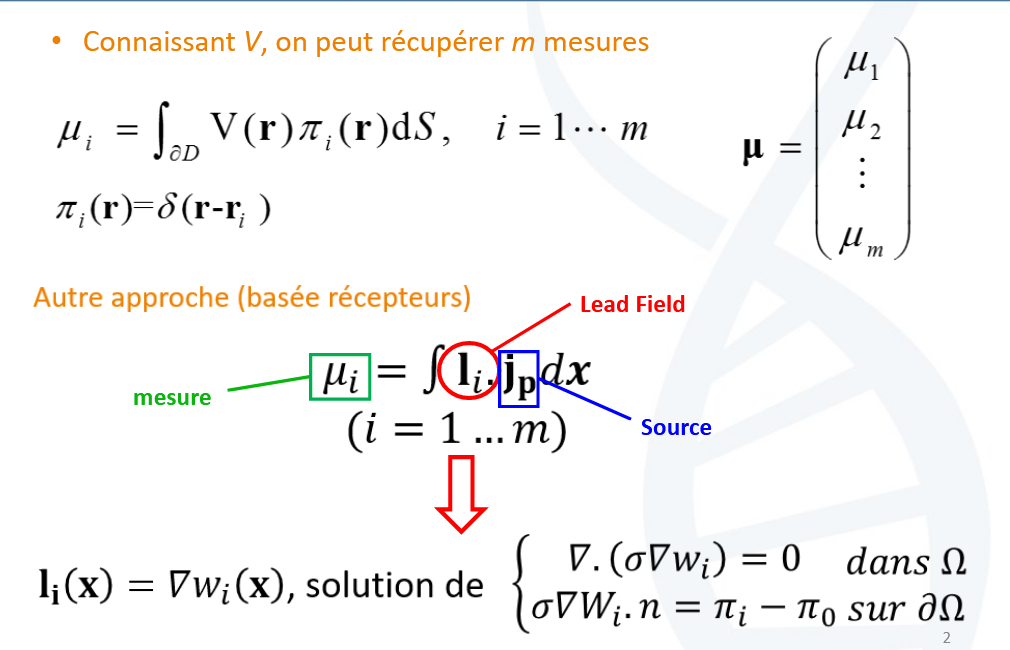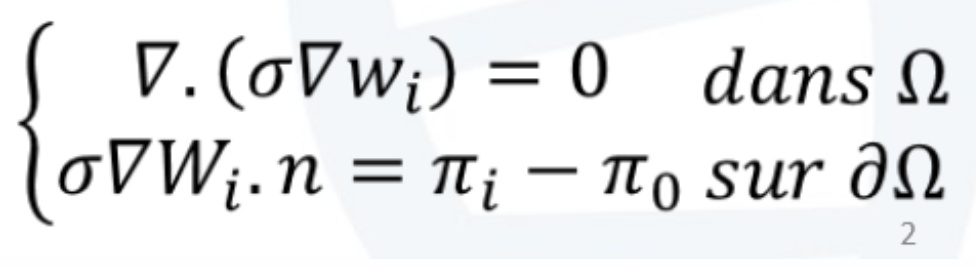# Forward problem - MEF

Hello,
part of my internship consists in solving the direct problem by the finite element method. I want to use DUNEuro, but I don't know if it solves this problem with one of these ways (in the picture).I'm sorry for my english.

thank you,

1 Like

Hi all,
The following is the formulation of the forward problem, known as the Poisson's Equation, with some hidden definition:In this equation, the Wi is the unknown, and in EEG it refers to the electrical potential or the "Voltage".
Brainstorm can solve this problem by different approachs as explained under the "Head Model" tutorial: https://neuroimage.usc.edu/brainstorm/Tutorials/HeadModel

You can also check this paper: Mosher et al 1999: https://pubmed.ncbi.nlm.nih.gov/10097460/

Depending on the available information on the subject (individual MRI data, DWI) and the desired precision, you can select among the methods:
-Analytical formulation (3shell) where the head is approximated by 3 concentric spheres.
with isotropic tissue conductivity for each layer.
-Boundary element method (BEM / Method des Element de Frontières), that uses the smoothed surface of the main tissues (brain, skull, and scalp) that can be obtained from the MRI
-The FEM, that uses the realistic head volume obtained from the MRI with the possibilities to simulate the tissues anisotropy.

The solution of this problem gives the lead field matrix (or the Gain matrix) which is defined by Ii(x) in your picture.

You can check further interesting discussions here about the LF or Gain matrix:

Regarding the FEM. It discretizes Poisson's equation and solves the weak formulation (simplified formulation) where the Wi is computed on all the nodes of the mesh, and for the EEG, we select the nearest node to the surface electrodes.